Add. Express each sum in simplest form.

Example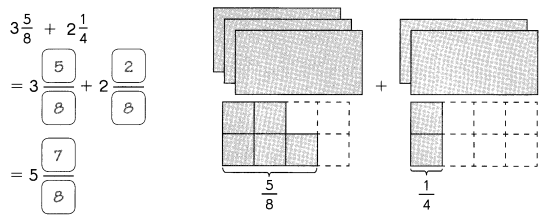Question 1.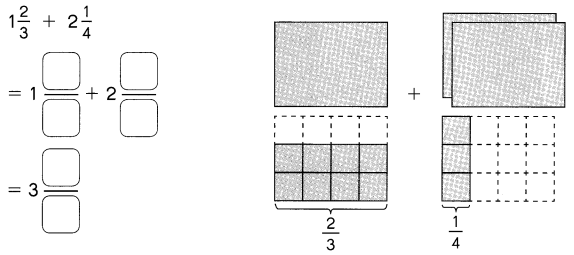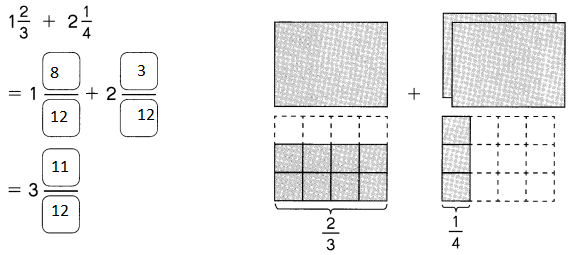Explanation:
Made the unlike denominators to like denominators

Question 2.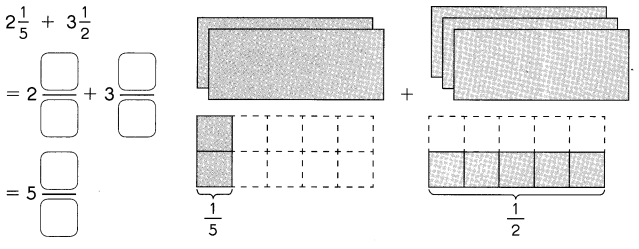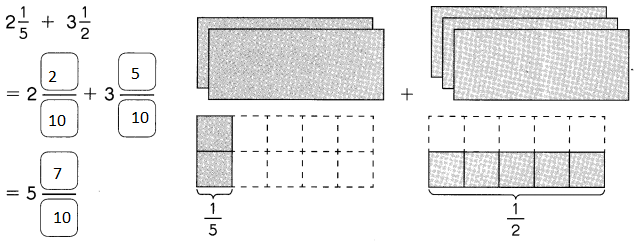Explanation:
Made the unlike denominators to like denominators

Add. Express each sum in simplest form

Question 3.
3$$\frac{2}{7}$$ + 2$$\frac{5}{14}$$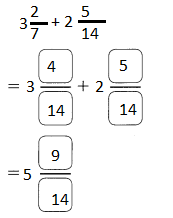Explanation:
Made the unlike denominators to like denominators

Question 4.
5$$\frac{7}{12}$$ + 3$$\frac{1}{4}$$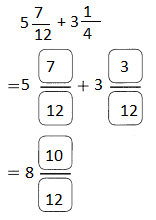Explanation:
Made the unlike denominators to like denominators

Question 5.
4$$\frac{1}{15}$$ + 1$$\frac{3}{10}$$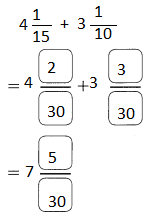Explanation:
Made the unlike denominators to like denominators

Question 6.
12$$\frac{1}{9}$$ + 9$$\frac{5}{6}$$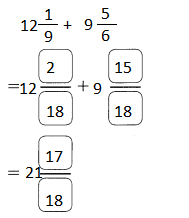Explanation:
Made the unlike denominators to like denominators

Add. Express each sum in simplest form.

Question 7.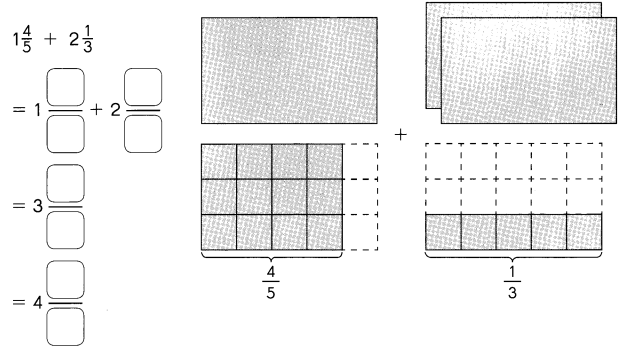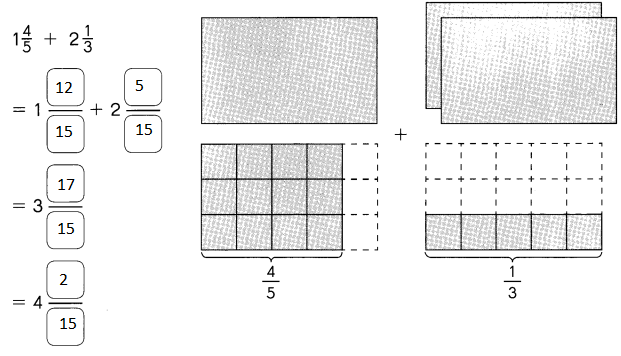Explanation:
Made the unlike denominators to like denominators

Question 8.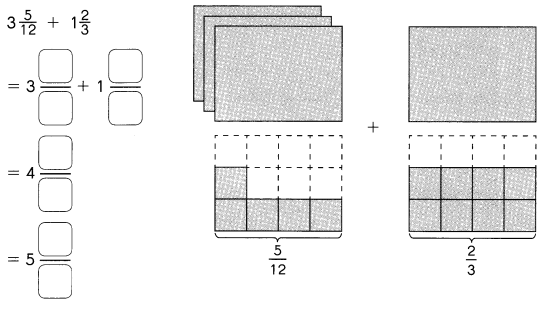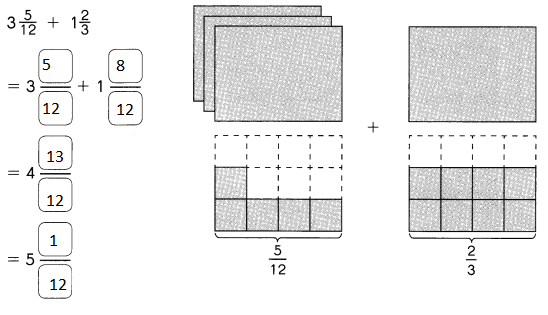Explanation:
Made the unlike denominators to like denominators

Question 9.
2$$\frac{3}{4}$$ + 3$$\frac{2}{5}$$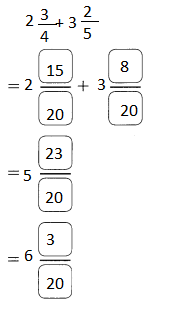Explanation:
Made the unlike denominators to like denominators

Question 10.
2$$\frac{5}{9}$$ + 1$$\frac{5}{6}$$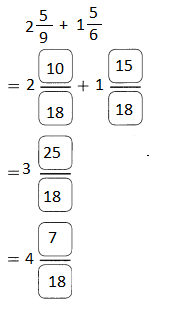Explanation:
Made the unlike denominators to like denominators

Question 11.
7$$\frac{8}{9}$$ + 9$$\frac{5}{12}$$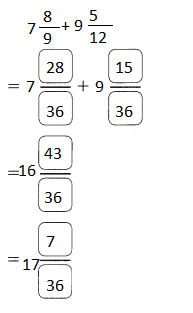Explanation:
Made the unlike denominators to like denominators

Question 12.
5$$\frac{7}{12}$$ + 1$$\frac{3}{4}$$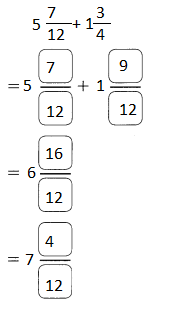Explanation:
Made the unlike denominators to like denominators

Use benchmarks to estimate each sum.

Example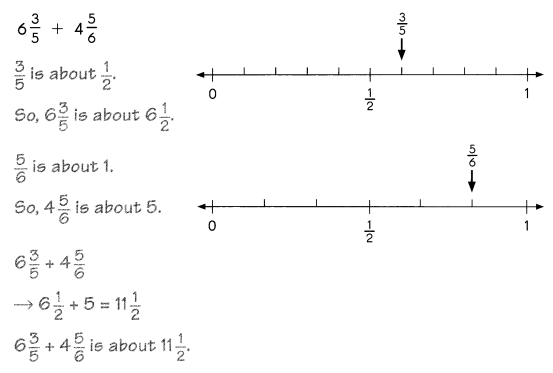Question 13.
9$$\frac{6}{7}$$ + 7$$\frac{5}{12}$$
17$$\frac{1}{2}$$
Explanation:
$$\frac{6}{7}$$ is near to the benchmark 1
$$\frac{5}{12}$$ is near to the bench mark $$\frac{1}{2}$$
9 + 1 = 10
10 + 7 $$\frac{1}{2}$$
17$$\frac{1}{2}$$

Question 14.
4$$\frac{7}{12}$$ + 10$$\frac{1}{9}$$
14 $$\frac{1}{2}$$
$$\frac{7}{12}$$ is near to the benchmark $$\frac{1}{2}$$
$$\frac{1}{12}$$ is near to the bench mark 0
4$$\frac{1}{2}$$ + 10
= 14 $$\frac{1}{2}$$